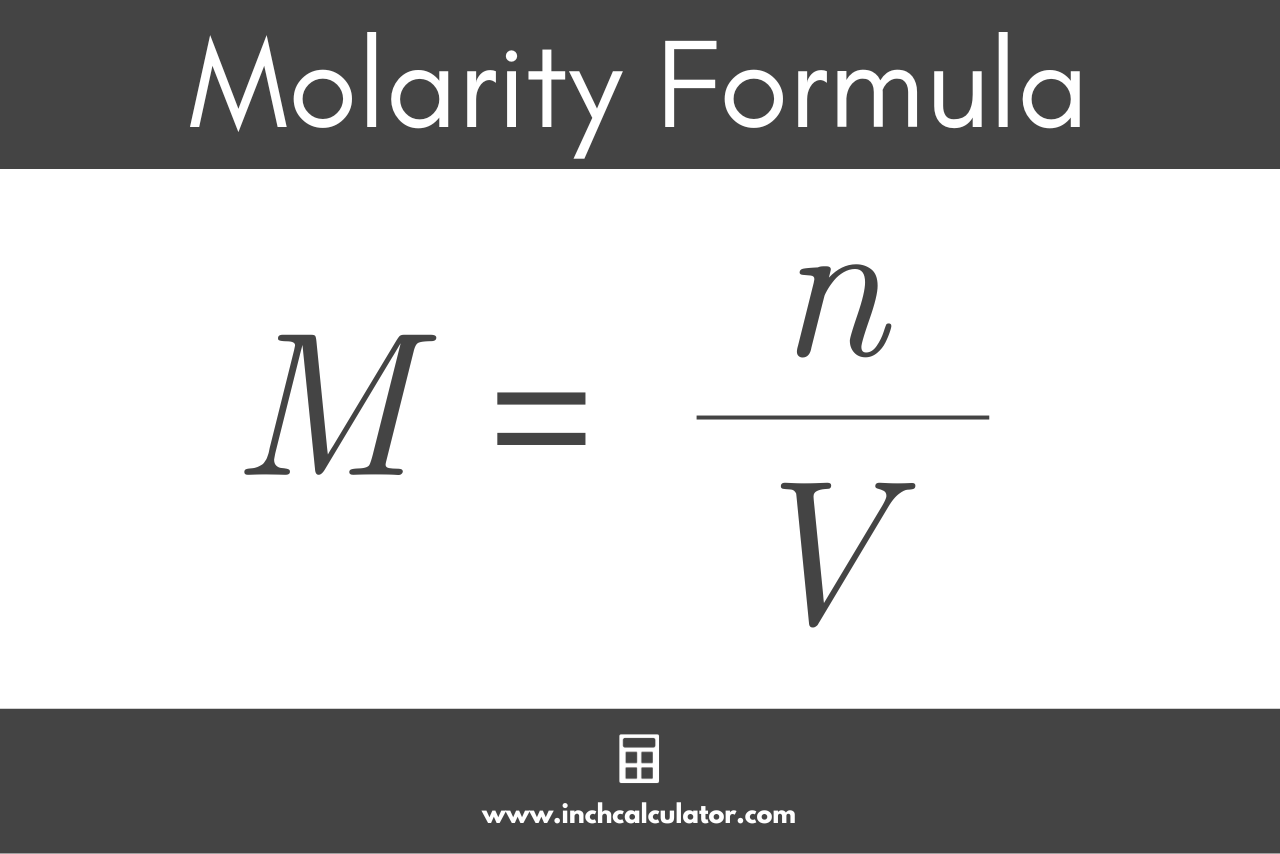# Molarity Calculator

Calculate the molarity, mass, or volume of a solution using the calculator below.

g/mol
g/mol
g/mol

## Molarity:

M
Learn how we calculated this below

## How to Calculate Molarity

Molarity is the ratio of the amount of solute (a molecule or compound) in moles dissolved in a volume of solution in liters. Molarity is also known as the molar concentration of a solution and is denoted using the letter M or (mol/L).

Molarity is commonly used to describe solutions of chemicals in a laboratory, because most chemical equations are written in units of moles. For example, if you need 2 moles of a chemical, and the chemical is in a 1M solution, then you know you need 2 L of that solution.

Molarity differs from molality in that molality is the ratio of the amount of the solute in moles to the mass of the solution in kg.

### Molarity Formula

You can find molarity using the following formula:

molarity (mol/L) = mass (g) ÷ molar mass (g/mol) ÷ volume (L)

The molarity is just the mass (g) divided by the molar mass (g/mol) divided by the volume (L). This formula can be simplified to the following standard equation, which is most commonly referenced when calculating molarity.

M = n / V

Thus, the molarity M of a solution (mol/L) is equal to the amount of solute n (mol) divided by the volume of substance V (L).The amount of the substance in moles can be found using the molar mass of the formula and the mass of the substance. Use our molar mass calculator if you need to calculate the molecular weight of the solute.

n = m / M

The amount of the substance n (mol) is equal to the mass of the substance m (g) divided by the molar mass M (g/mol).

Of course, you can also use our mole calculator to find the amount of the substance in moles.

## How to Calculate Mass

To find the mass of a solution, we can rearrange the formula above:

mass (g) = molarity (mol/L) × volume (L) × molar mass (g/mol)

The mass in grams of a substance in solution is equal to the molarity in mol/L times the volume in liters times the molar mass of the substance in g/mol.

## How to Calculate Volume

Similarly, to find the volume of a solution, we also use a formula:

volume (L) = mass (g) ÷ molar mass (g/mol) ÷ molarity (mol/L)

The volume (L) of a solution is equal to the mass (g) divided by the molar mass of the substance (g/mol), divided by the molarity (mol).